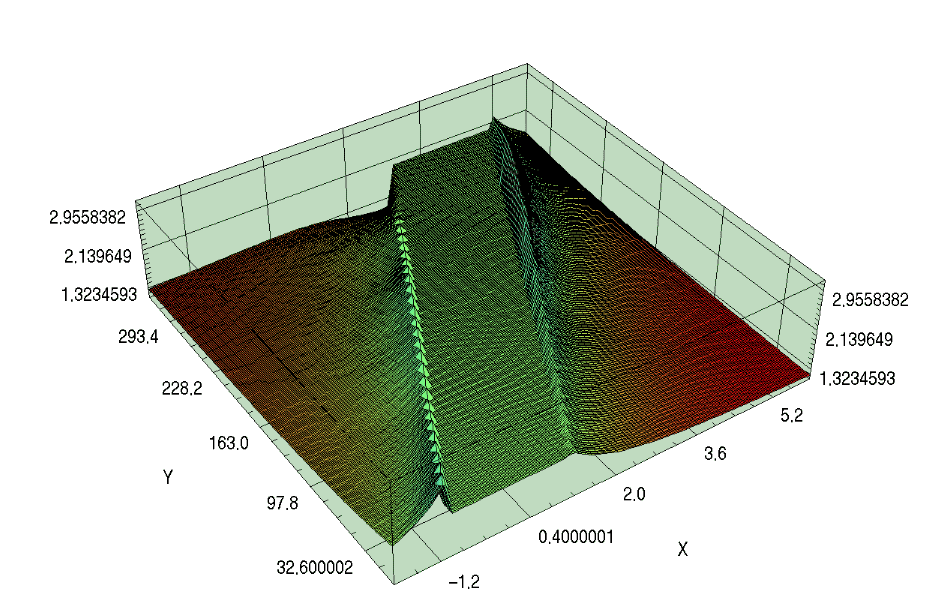Evolving Boosted Black Holes

The following is a short summary of recent results from an evolution of a boosted Schwarzschild black hole. The initial data for a single moving hole was obtained taking the spacetime metric for a Schwarzschild black hole in ingoing Eddington-Finkelstein coordinates and boosting it in the z-direction. The resulting spacetime metric is then taken and decomposed into a 3-metric, extrinisic curvature, lapse and shift vector. The 3-metric and Extrinsic curvature are used for initial data and the lapse and shift, which are now a function of coordinate time are utilized during the course of the evolution. That is, the lapse and shift are set exactly. This seems to be compatible with using Dirichlet outer boundary conditions, where we set data from exact analytic time-dependent expressions for the 3-metric and extrinsic curvature at the outer boundaries. The black hole inner boundary for these runs is placed 4 grid zones from the apparent horizon and one-sided differencing is carried out. The one-sided differencing is O(h^2), where h is the grid-spacing which is uniform in all directions. The difference stencils are computed such that the resulting truncation error is matched to leading order to the interior centered differencing trunction error.

As the evolution proceeds the black hole moves in the z-direction and the region of the black hole that is excised follows it. The important point to note here is that this the first sucessful moving black hole evolution that we know of utilizing black hole excision. Now, as the excised region, defined by the mask (1 where defined, 0 where undefined) moves through the computational domain a problem arises. Points that were previously inside the excised region now appear in the computational domain with no "correct" value ascribed to them for their field values. These points obviously appear at the trailing edge of the black hole whereas points to the leading edge get "masked out" as they fall into the hole. The new points that appear in domain are updated causally using Causal Differencing and hence pose no real problem. The following shows excerpts of a recent run where the black hole was boosted in the z-direction with a boost velocity of 0.1 where c=1. The grid size used here was 33x33x65 with spatial extents -2<=x<=2 -2<=y<=2 and -2<=z<=6(h=1/8). The Courant factor used for this run was 0.25 and the hole radius was 1.5 or M=0.75. The inner boundary was placed 3points from the horizon. The black hole started at an initial location of (0,0,0) and stopped at t~54M and a grid location of z~4.0 or ~5.4M. This meant that the black hole propagated over one diameter with the points left behind settling down. The instability/coordinate effect that stopped the run was around the trailing edge of the black hole.

The following shows a spacetime picture of g_rr versus time and z. The axis labelled X represents the z-axis and the axis labelled Y represents time in grid coordinates. The vertical axis is g_rr. The flat region in between is the excised region.An mpeg movie of the 1D dump that the above plot was created from is available at this link. The movie shows g_rr versus z in time.

This run stopped due to an instability at t~54M. A similar run with 33x33x97 grid size also stopped at about the same time. The code crash is not due to the black hole running into the other boundary. A similar 33x33x65 with Courant factor 0.125 ran up to ~42M. The instability/coordinate stretching notwithstanding, these runs have demonstrated that it is possible to evolve moving black holes utilizing existing infrastructure.

The adm.in parameter file and the I/O file, outlist.in are available. Data from these runs can be obtained from the data vault.

mijan@galileo.ph.utexas.edu